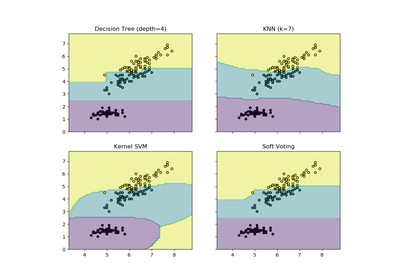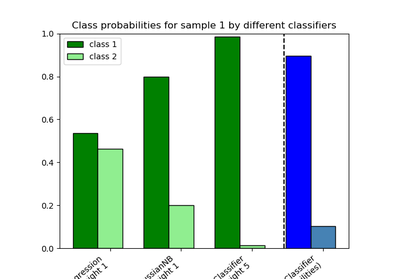# `sklearn.ensemble`.VotingClassifier¶

class `sklearn.ensemble.``VotingClassifier`(estimators, voting=’hard’, weights=None, n_jobs=1, flatten_transform=None)[source]

Soft Voting/Majority Rule classifier for unfitted estimators.

New in version 0.17.

Read more in the User Guide.

Parameters: estimators : list of (string, estimator) tuples Invoking the `fit` method on the `VotingClassifier` will fit clones of those original estimators that will be stored in the class attribute `self.estimators_`. An estimator can be set to None using `set_params`. voting : str, {‘hard’, ‘soft’} (default=’hard’) If ‘hard’, uses predicted class labels for majority rule voting. Else if ‘soft’, predicts the class label based on the argmax of the sums of the predicted probabilities, which is recommended for an ensemble of well-calibrated classifiers. weights : array-like, shape = [n_classifiers], optional (default=`None`) Sequence of weights (float or int) to weight the occurrences of predicted class labels (hard voting) or class probabilities before averaging (soft voting). Uses uniform weights if None. n_jobs : int, optional (default=1) The number of jobs to run in parallel for `fit`. If -1, then the number of jobs is set to the number of cores. flatten_transform : bool, optional (default=None) Affects shape of transform output only when voting=’soft’ If voting=’soft’ and flatten_transform=True, transform method returns matrix with shape (n_samples, n_classifiers * n_classes). If flatten_transform=False, it returns (n_classifiers, n_samples, n_classes). estimators_ : list of classifiers The collection of fitted sub-estimators as defined in `estimators` that are not None. classes_ : array-like, shape = [n_predictions] The classes labels.

Examples

```>>> import numpy as np
>>> from sklearn.linear_model import LogisticRegression
>>> from sklearn.naive_bayes import GaussianNB
>>> from sklearn.ensemble import RandomForestClassifier, VotingClassifier
>>> clf1 = LogisticRegression(random_state=1)
>>> clf2 = RandomForestClassifier(random_state=1)
>>> clf3 = GaussianNB()
>>> X = np.array([[-1, -1], [-2, -1], [-3, -2], [1, 1], [2, 1], [3, 2]])
>>> y = np.array([1, 1, 1, 2, 2, 2])
>>> eclf1 = VotingClassifier(estimators=[
...         ('lr', clf1), ('rf', clf2), ('gnb', clf3)], voting='hard')
>>> eclf1 = eclf1.fit(X, y)
>>> print(eclf1.predict(X))
[1 1 1 2 2 2]
>>> eclf2 = VotingClassifier(estimators=[
...         ('lr', clf1), ('rf', clf2), ('gnb', clf3)],
...         voting='soft')
>>> eclf2 = eclf2.fit(X, y)
>>> print(eclf2.predict(X))
[1 1 1 2 2 2]
>>> eclf3 = VotingClassifier(estimators=[
...        ('lr', clf1), ('rf', clf2), ('gnb', clf3)],
...        voting='soft', weights=[2,1,1],
...        flatten_transform=True)
>>> eclf3 = eclf3.fit(X, y)
>>> print(eclf3.predict(X))
[1 1 1 2 2 2]
>>> print(eclf3.transform(X).shape)
(6, 6)
>>>
```

Methods

 `fit`(X, y[, sample_weight]) Fit the estimators. `fit_transform`(X[, y]) Fit to data, then transform it. `get_params`([deep]) Get the parameters of the VotingClassifier `predict`(X) Predict class labels for X. `score`(X, y[, sample_weight]) Returns the mean accuracy on the given test data and labels. `set_params`(**params) Setting the parameters for the voting classifier `transform`(X) Return class labels or probabilities for X for each estimator.
`__init__`(estimators, voting=’hard’, weights=None, n_jobs=1, flatten_transform=None)[source]
`fit`(X, y, sample_weight=None)[source]

Fit the estimators.

Parameters: X : {array-like, sparse matrix}, shape = [n_samples, n_features] Training vectors, where n_samples is the number of samples and n_features is the number of features. y : array-like, shape = [n_samples] Target values. sample_weight : array-like, shape = [n_samples] or None Sample weights. If None, then samples are equally weighted. Note that this is supported only if all underlying estimators support sample weights. self : object
`fit_transform`(X, y=None, **fit_params)[source]

Fit to data, then transform it.

Fits transformer to X and y with optional parameters fit_params and returns a transformed version of X.

Parameters: X : numpy array of shape [n_samples, n_features] Training set. y : numpy array of shape [n_samples] Target values. X_new : numpy array of shape [n_samples, n_features_new] Transformed array.
`get_params`(deep=True)[source]

Get the parameters of the VotingClassifier

Parameters: deep: bool : Setting it to True gets the various classifiers and the parameters of the classifiers as well
`predict`(X)[source]

Predict class labels for X.

Parameters: X : {array-like, sparse matrix}, shape = [n_samples, n_features] Training vectors, where n_samples is the number of samples and n_features is the number of features. maj : array-like, shape = [n_samples] Predicted class labels.
`predict_proba`

Compute probabilities of possible outcomes for samples in X.

Parameters: X : {array-like, sparse matrix}, shape = [n_samples, n_features] Training vectors, where n_samples is the number of samples and n_features is the number of features. avg : array-like, shape = [n_samples, n_classes] Weighted average probability for each class per sample.
`score`(X, y, sample_weight=None)[source]

Returns the mean accuracy on the given test data and labels.

In multi-label classification, this is the subset accuracy which is a harsh metric since you require for each sample that each label set be correctly predicted.

Parameters: X : array-like, shape = (n_samples, n_features) Test samples. y : array-like, shape = (n_samples) or (n_samples, n_outputs) True labels for X. sample_weight : array-like, shape = [n_samples], optional Sample weights. score : float Mean accuracy of self.predict(X) wrt. y.
`set_params`(**params)[source]

Setting the parameters for the voting classifier

Valid parameter keys can be listed with get_params().

Parameters: params: keyword arguments : Specific parameters using e.g. set_params(parameter_name=new_value) In addition, to setting the parameters of the `VotingClassifier`, the individual classifiers of the `VotingClassifier` can also be set or replaced by setting them to None.

Examples

# In this example, the RandomForestClassifier is removed clf1 = LogisticRegression() clf2 = RandomForestClassifier() eclf = VotingClassifier(estimators=[(‘lr’, clf1), (‘rf’, clf2)] eclf.set_params(rf=None)

`transform`(X)[source]

Return class labels or probabilities for X for each estimator.

Parameters: X : {array-like, sparse matrix}, shape = [n_samples, n_features] Training vectors, where n_samples is the number of samples and n_features is the number of features. If `voting=’soft’` and `flatten_transform=True`: : array-like = (n_classifiers, n_samples * n_classes) otherwise array-like = (n_classifiers, n_samples, n_classes) Class probabilities calculated by each classifier. If `voting=’hard’`: : array-like = [n_samples, n_classifiers] Class labels predicted by each classifier.

## Examples using `sklearn.ensemble.VotingClassifier`¶Plot the decision boundaries of a VotingClassifierPlot class probabilities calculated by the VotingClassifier